﻿ Explaining Feature Importance by example of a Random Forest – Data Science Austria

# Explaining Feature Importance by example of a Random ForestIn many (business) cases it is equally important to not only have an accurate, but also an interpretable model. Oftentimes, apart from wanting to know what our model’s house price prediction is, we also wonder why it is this high/low and which features are most important in determining the forecast. Another example might be predicting customer churn — it is very nice to have a model that is successfully predicting which customers are prone to churn, but identifying which variables are important can help us in early detection and maybe even improving the product/service!

Knowing feature importance indicated by machine learning models can benefit you in multiple ways, for example:

• by getting a better understanding of the model’s logic you can not only verify it being correct, but also work on improving the model by focusing only on the important variables
• the above can be used for variable selection — you can remove x variables that are not that significant and have similar or better performance in much shorter training time
• in some business cases it makes sense to sacrifice some accuracy for the sake of interpretability. For example, when a bank rejects a loan application, it must also have a reasoning behind the decision, which can also be presented to the customer

That is why in this article I would like to explore different approaches to interpreting feature importance by example of a Random Forest model. Most of them are also applicable to different models, starting from linear regression and ending with black-boxes such as XGBoost.

One thing to note is that the more accurate our model is, the more we can trust feature importance measures and other interpretations. I assume that the model we build is reasonably accurate (as each data scientist will strive to have such a model) and in this article I focus on importance measures.

### Data

For this example I will use the Boston house prices dataset (so a regression problem). But the approaches described in this article work just as well with classification problems, the only difference is the metric used for evaluation.

The only non-standard thing in preparing the data is the addition of a random column to the dataset. Logically, it has no predictive power over the dependent variable (Median value of owner-occupied homes in \$1000’s), so it should not be an important feature in the model. Let’s see how it will turn out.

Below I inspect the relationship between the random feature and the target variable. As it can be observed, there is no pattern on the scatterplot and the correlation is almost 0.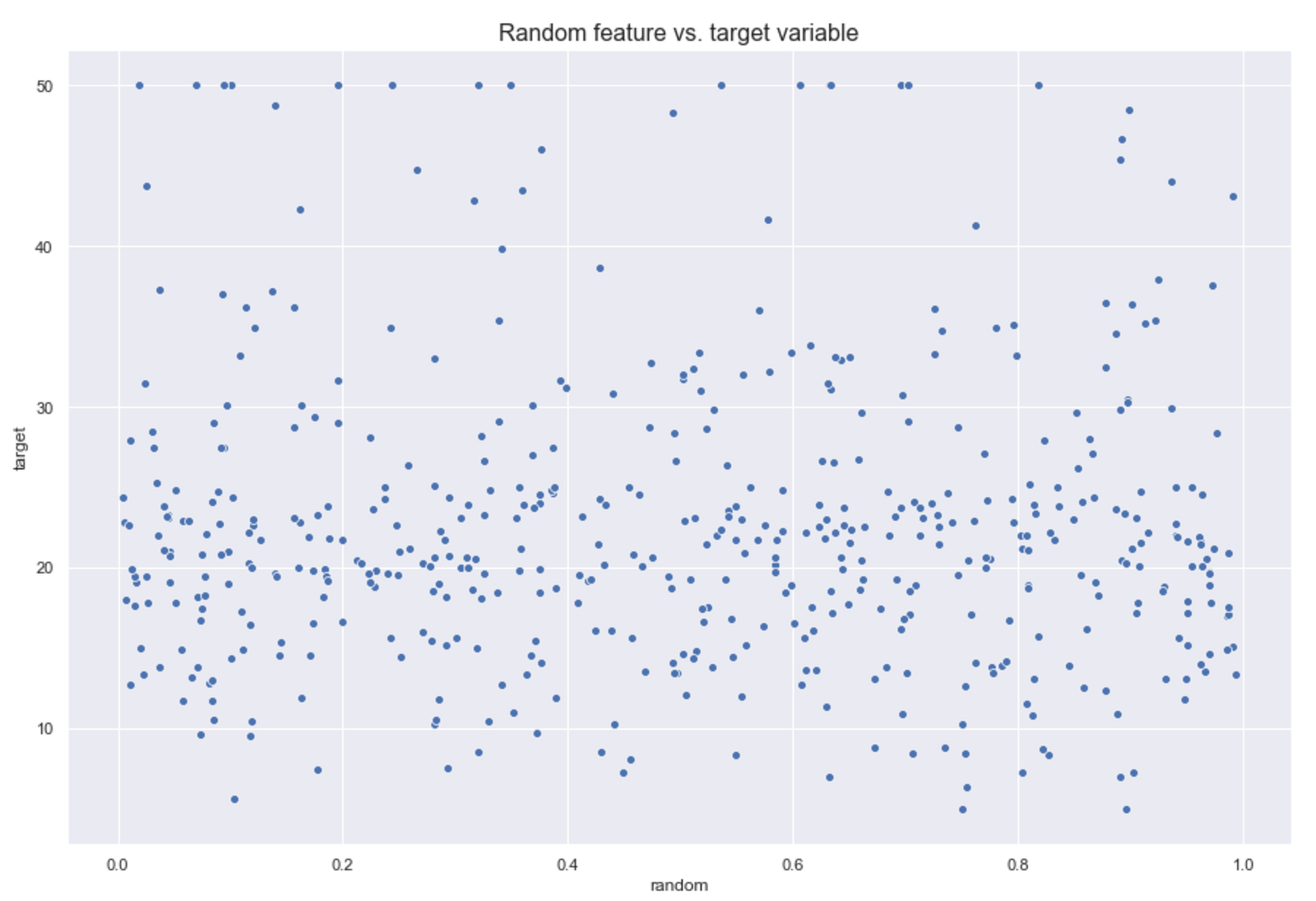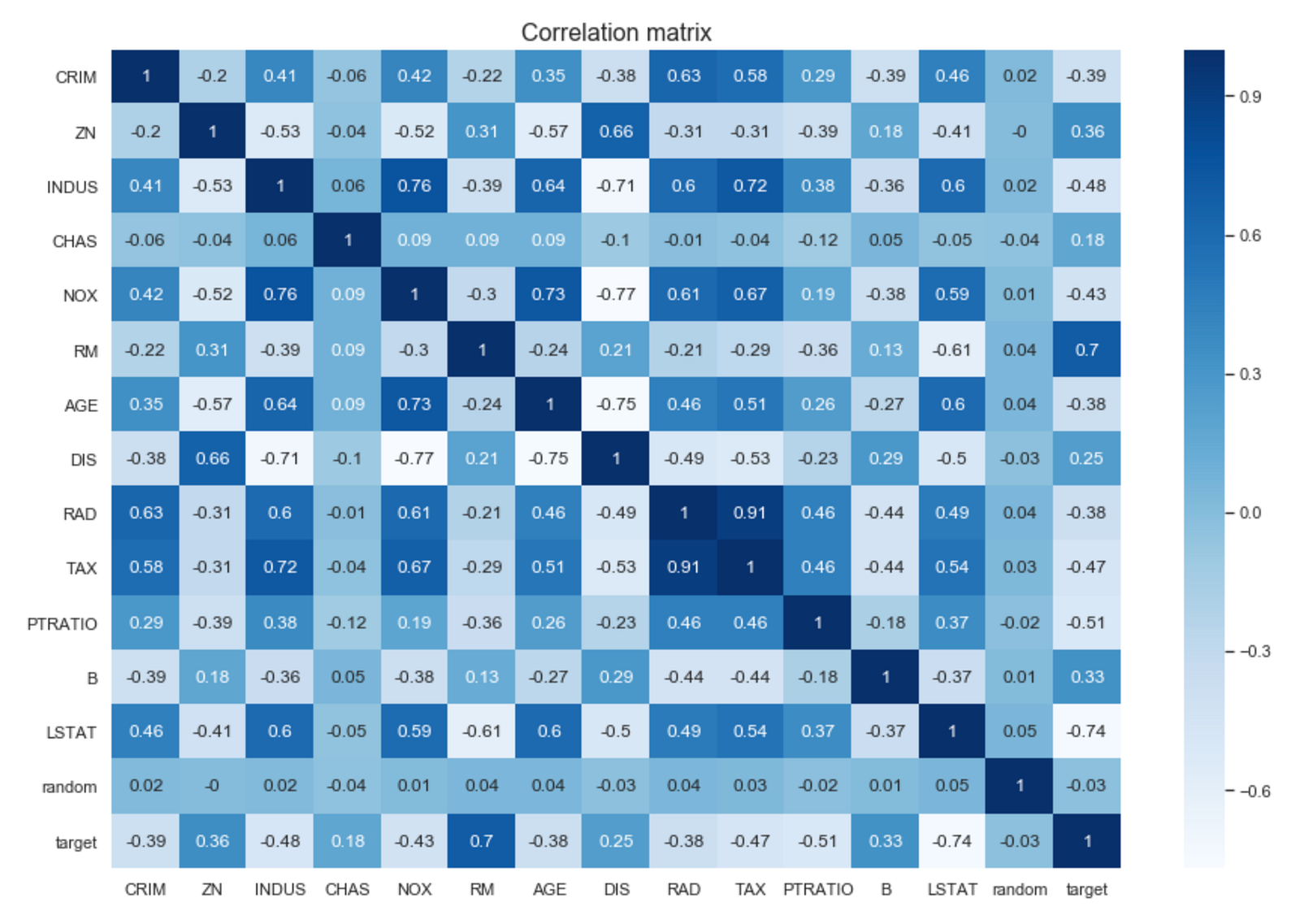One thing to note here is that there is not much sense in interpreting the correlation for `CHAS`, as it is a binary variable and different methods should be used for it.

### Benchmark model

I train a plain Random Forest model to have a benchmark. I set a `random_state` to ensure results comparability. I also use bootstrap and set `oob_score = True` so I could later use the out-of-bag error.

Briefly on the subject of out-of-bag error, each tree in the Random Forest is trained on a different dataset, sampled with replacement from the original data. This results in around ~2/3 of distinct observations in each training set. The out-of-bag error is calculated on all the observations, but for calculating each row’s error the model only considers trees which have not seen this row during training. This is similar to evaluating the model on a validation set. You can read more here.

```R^2 Training Score: 0.93 OOB Score: 0.58 R^2 Validation Score: 0.76
```

Well, there is some overfitting in the model, as it performs much worse on OOB sample and worse on the validation set. But let’s say it is good enough and move forward to feature importances (measured on the training set performance). Some of the approaches can also be used for validation/OOB sets, to gain further interpretability on the unseen data.

### 1. Overall feature importances

By overall feature importances I mean the ones derived at model level, i.e., saying that in a given model these features are most important in explaining the target variable.

### 1.1. Default Scikit-learn’s feature importances

Let’s start with decision trees to build some intuition. In decision trees, every node is a condition how to split values in a single feature, so that similar values of dependent variable end up in the same set after the split. The condition is based on impurity, which in case of classification problems is Gini impurity / information gain (entropy), while for regression trees its variance. So when training a tree we can compute how much each feature contributes to decreasing the weighted impurity. `feature_importances_` in Scikit-Learn is based on that logic, but in case of Random Forest we are talking about averaging the decrease in impurity over trees.

Pros:

• fast calculation
• easy to retrieve — one command

Cons:

• biased approach, as it has a tendency to inflate the importance of continuous features or high-cardinality categorical variables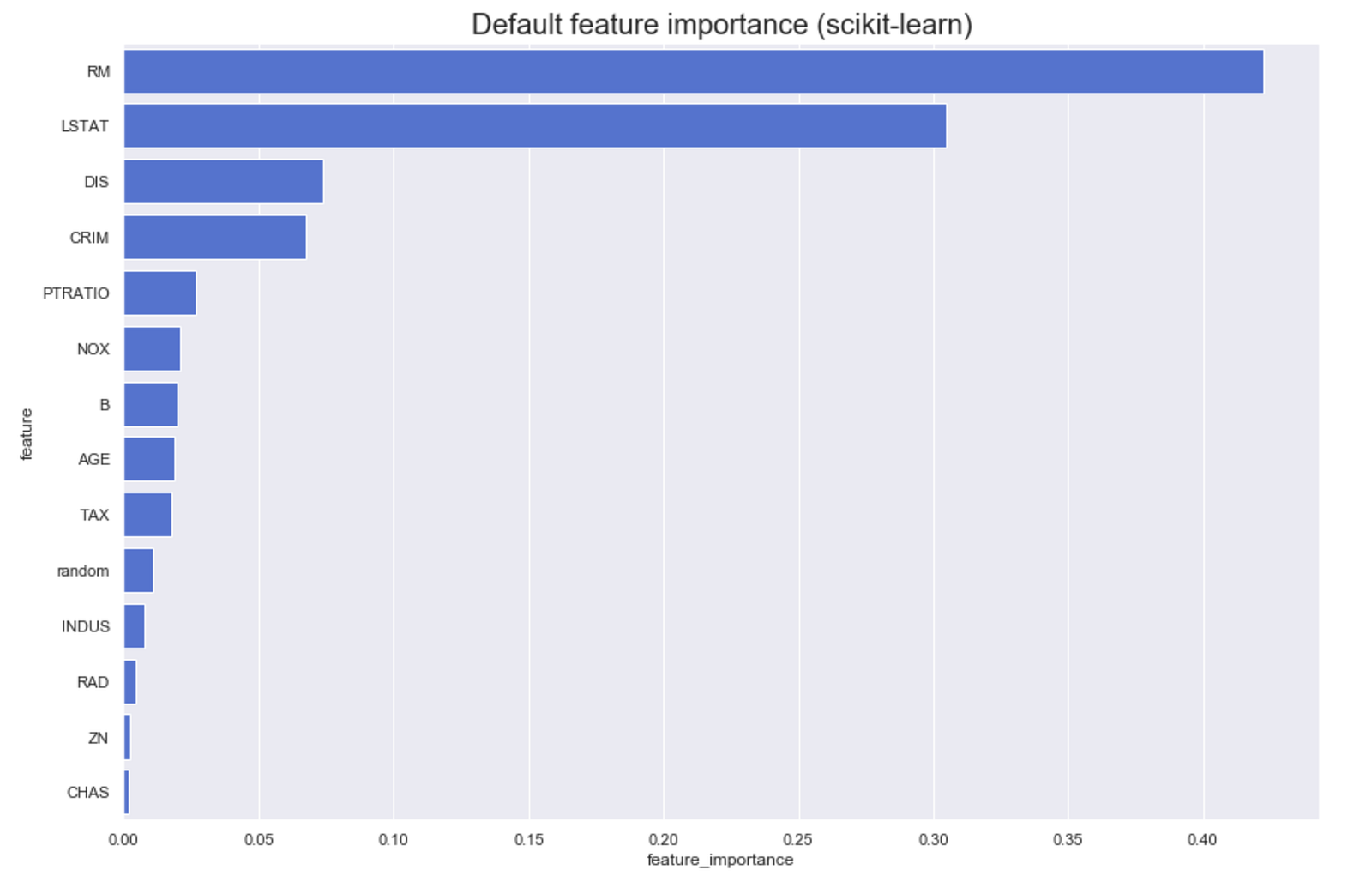It seems that the top 3 most important features are:

• average number of rooms
• % lower status of the population
• weighted distances to five Boston employment centres

What seems surprising though is that a column of random values turned out to be more important than:

• proportion of non-retail business acres per town
• index of accessibility to radial highways
• proportion of residential land zoned for lots over 25,000 sq.ft.
• Charles River dummy variable (= 1 if tract bounds river; 0 otherwise)

Intuitively this feature should have zero importance on the target variable. Let’s see how it is evaluated by different approaches.

### 1.2. Permutation feature importance

This approach directly measures feature importance by observing how random re-shuffling (thus preserving the distribution of the variable) of each predictor influences model performance.

The approach can be described in the following steps:

1. Train the baseline model and record the score (accuracy/R²/any metric of importance) by passing validation set (or OOB set in case of Random Forest). This can also be done on the training set, at the cost of sacrificing information about generalisation.
2. Re-shuffle values from one feature in the selected dataset, pass the dataset to the model again to obtain predictions and calculate the metric for this modified dataset. The feature importance is the difference between the benchmark score and the one from the modified (permuted) dataset.
3. Repeat 2. for all feature in the dataset.

Pros:

• applicable to any model
• reasonably efficient
• reliable technique
• no need to retrain the model at each modification of the dataset

Cons:

• more computationally expensive than default `feature_importances`
• permutation importance overestimates the importance of correlated predictors — Strobl et al (2008)

As for the second problem with this method, I have already plotted the correlation matrix above. However, I will use a function from one of the libraries I use to visualise Spearman’s correlations. The difference between standard Pearson’s correlation is that this one first transforms variables into ranks and only then runs Pearson’s correlation on the ranks.

Spearman’s correlation:

• is nonparametric
• does not assume linear relationship between variables
• it looks for monotonic relationships.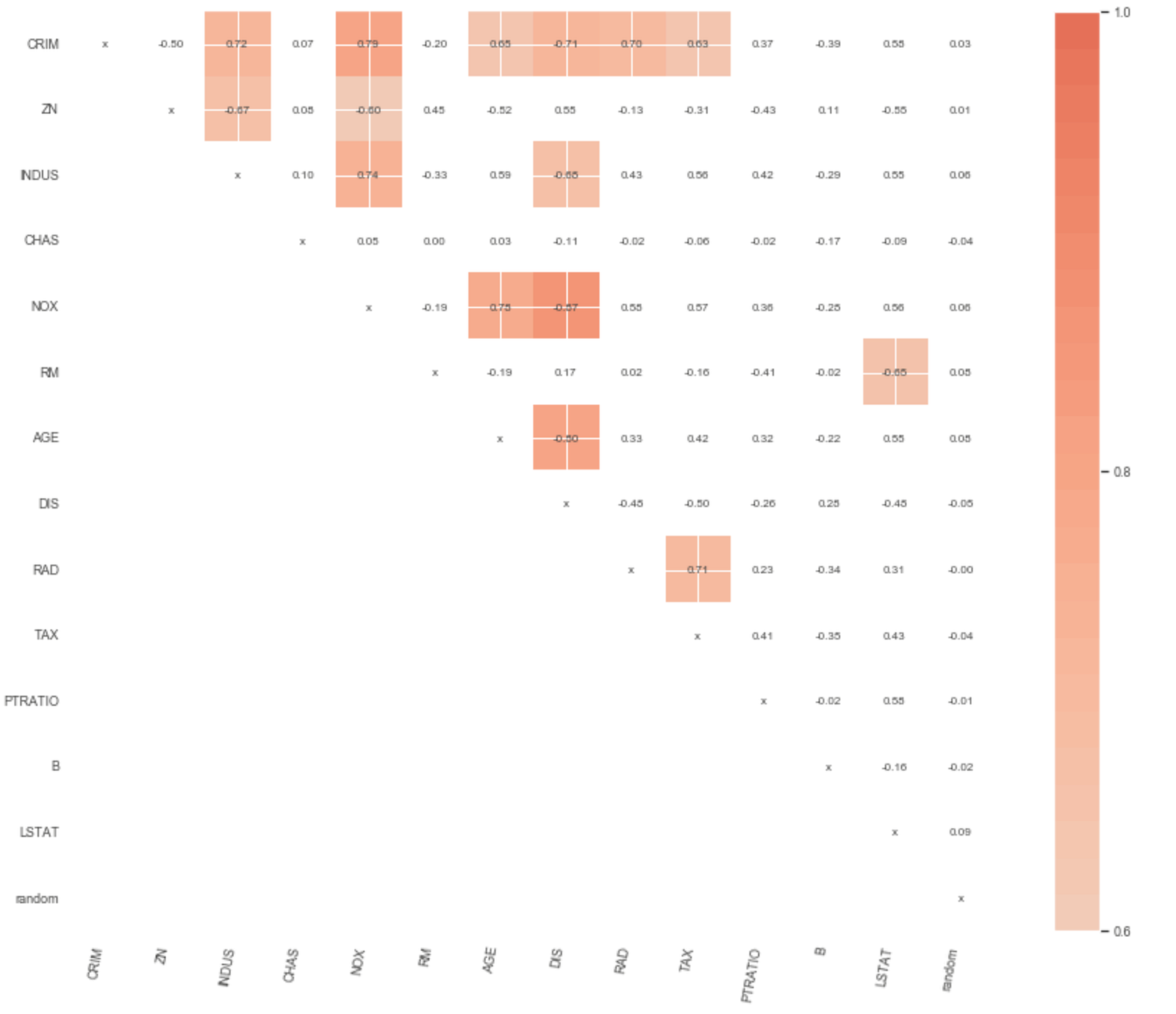I found two libraries with this functionality, not that it is difficult to code it. Let’s go over both of them as they have some unique features.

`rfpimp`

One thing to note about this library is that we have to provide a metric as a function of the form `metric(model, X, y)`. This way we can use more advanced approaches such as using the OOB score of Random Forest. This library already contains functions for that (`oob_regression_r2_score)`. But to keep the approach uniform, I will calculate the metrics on the training set (losing information about generalisation).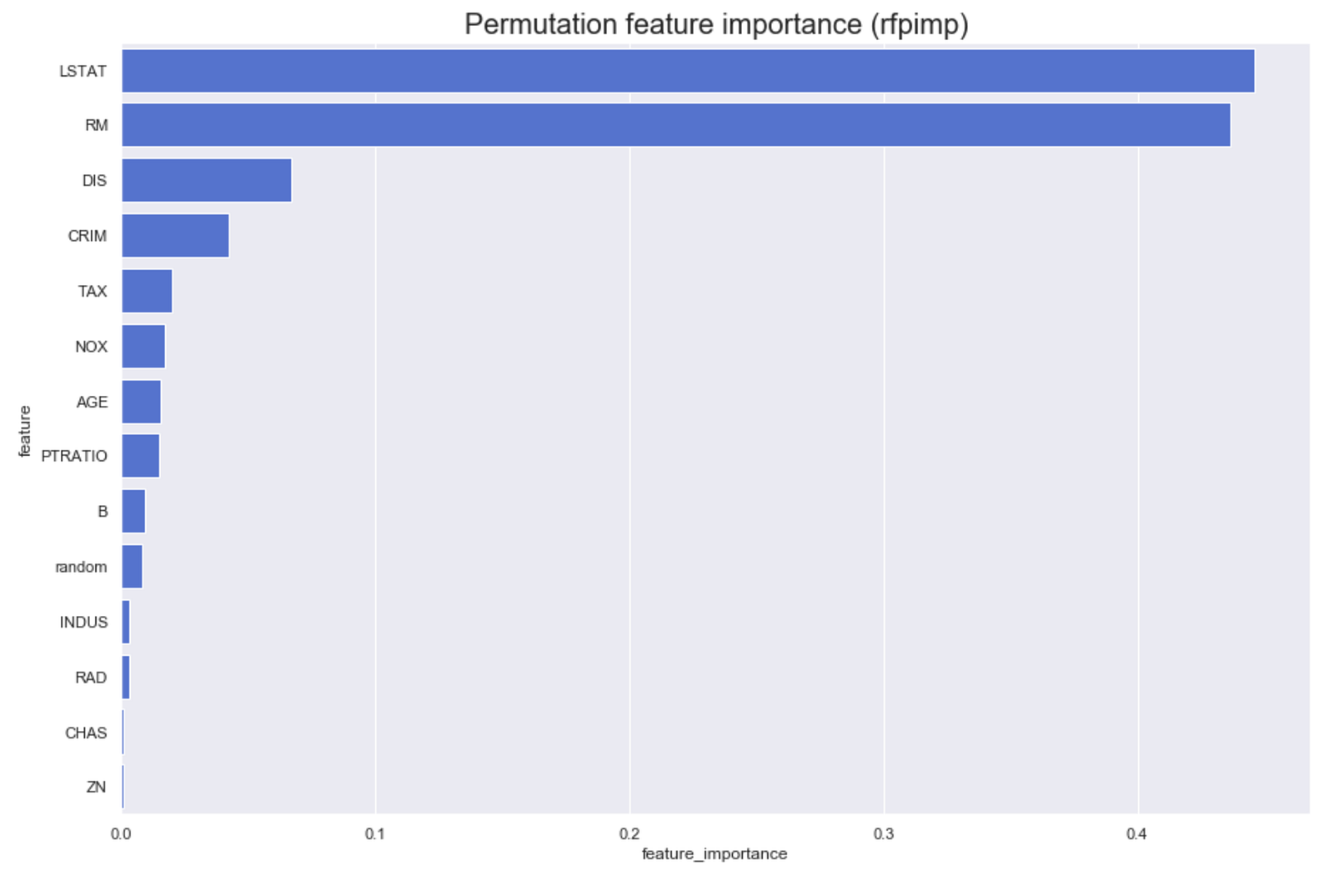The plot confirms what we have seen above, that 4 variables are less important than a random variable! Surprising… The top 4 stayed the same though. One more nice feature about `rfpimp` is that it contains functionalities for dealing with the issue of collinear features (that was the idea behind showing the Spearman’s correlation matrix). For brevity I will not show this case here, but you can read more in this great [article](https://explained.ai/rf-importance/index.html#5) by the authors of the library.

`eli5`

There are a few differences from the basic approach of `rfpimp` and the one employed in `eli5`. Some of them are:

• there are parameters `cv` and `refit` connected to using cross-validation. In this example I set them to `None`, as I do not use it but it might come in handy in some cases.
• there is a `metric` parameter, which as in `rfpimp` accepts a function in the form of `metric(model, X, y)`. If this parameter is not specified, the function will use the default `score` method of the estimator.
• `n_iter` – number of random shuffle iterations, the end score is the average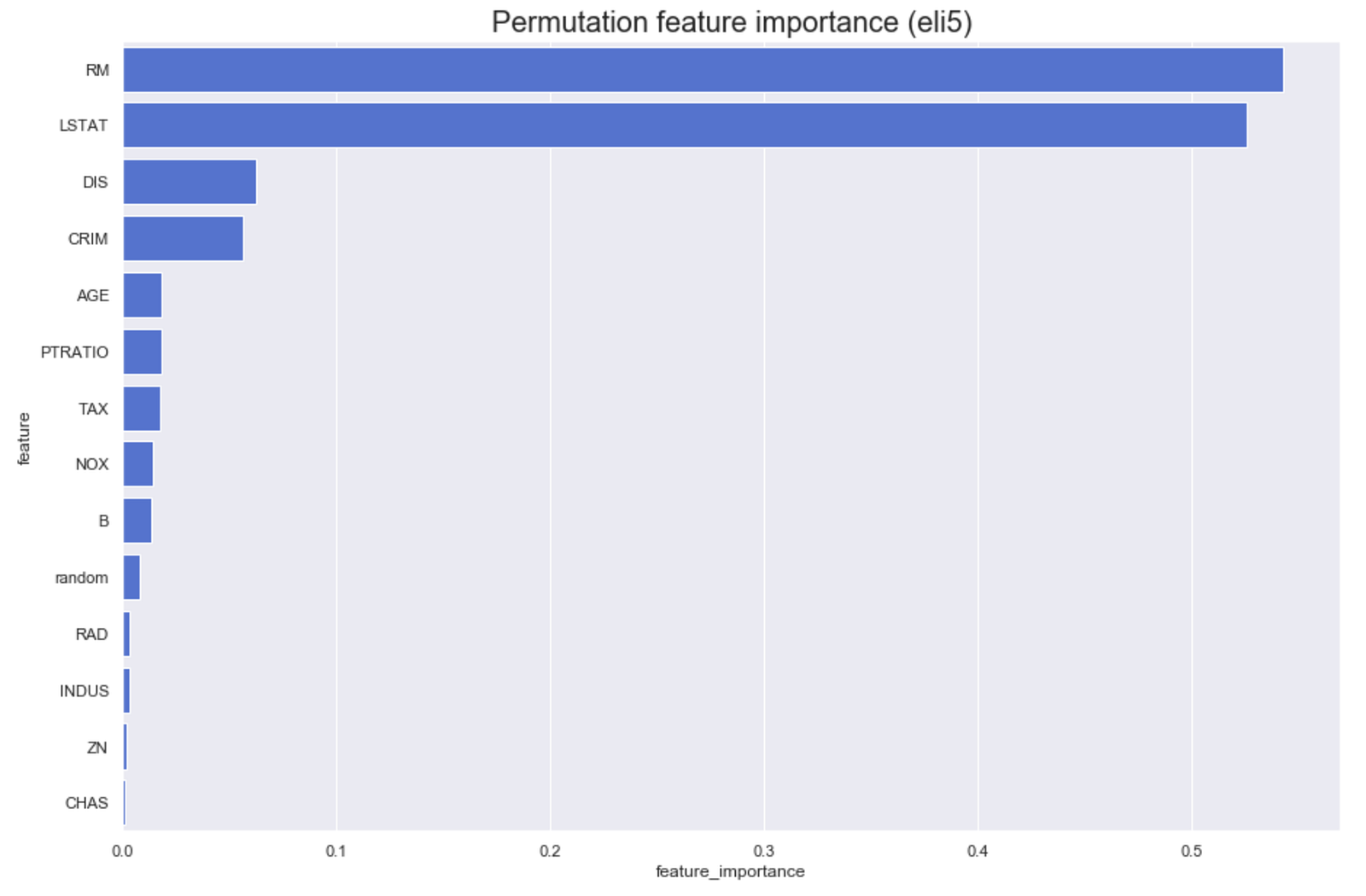The results are very similar to the previous ones, even as these came from multiple reshuffles per column. One extra nice thing about `eli5` is that it is really easy to use the results of permutation approach to carry out feature selection by using Scikit-learn’s `SelectFromModel` or `RFE`.

### 1.3. Drop Column feature importance

This approach is quite an intuitive one, as we investigate the importance of a feature by comparing a model with all features versus a model with this feature dropped for training.

I created a function (based on `rfpimp`‘s implementation) for this approach below, which shows the underlying logic.

Pros:

• most accurate feature importance

Cons:

• potentially high computation cost due to retraining the model for each variant of the dataset (after dropping a single feature column)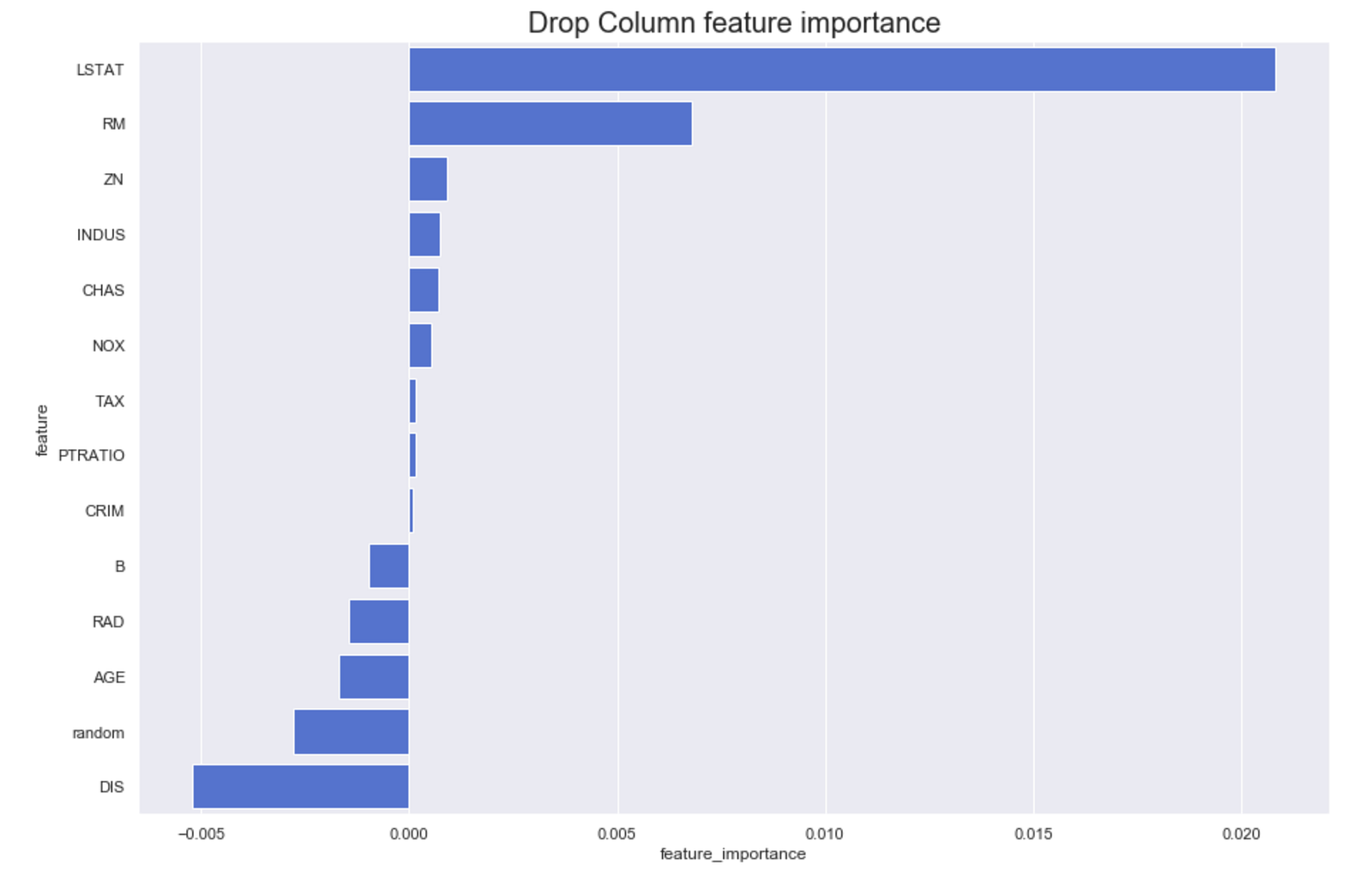Here it gets interesting. First of all, negative importance in this case means that removing a given feature from the model actually improves the performance. So this is nice to see in case of `random`, but what is weird is that the highest performance boost can be observed after removing `DIS`, which was the third most important variable in previous approaches. Unfortunately I do not have a good explanation for this. If you have any ideas please let me know in the comments!

### 2. Observation level feature importance

By observation level feature importances I mean ones that had most impact on explaining a particular observation fed to the model. For example, in case of credit scoring, we would be able to say that these features had most impact on determining client’s credit score.

### 2.1. Treeinterpreter

The main idea of `treeinterpreter` is that it uses the underlying trees in Random Forest to explain how each feature contributes the end value. We can observe how the value of the prediction (defined as the sum of each feature contributions + average given by the initial node that is based on the entire training set) changes along the prediction path within the decision tree (after every split), together with the information which feature caused the split (so also the change in prediction).

The formula for the prediction function (f(x)) can be written down as: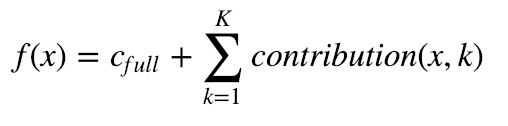where c_full is the average of the entire dataset (initial node), K is the total number of features.

This may sound complicated, but take a look at an example from the author of the library: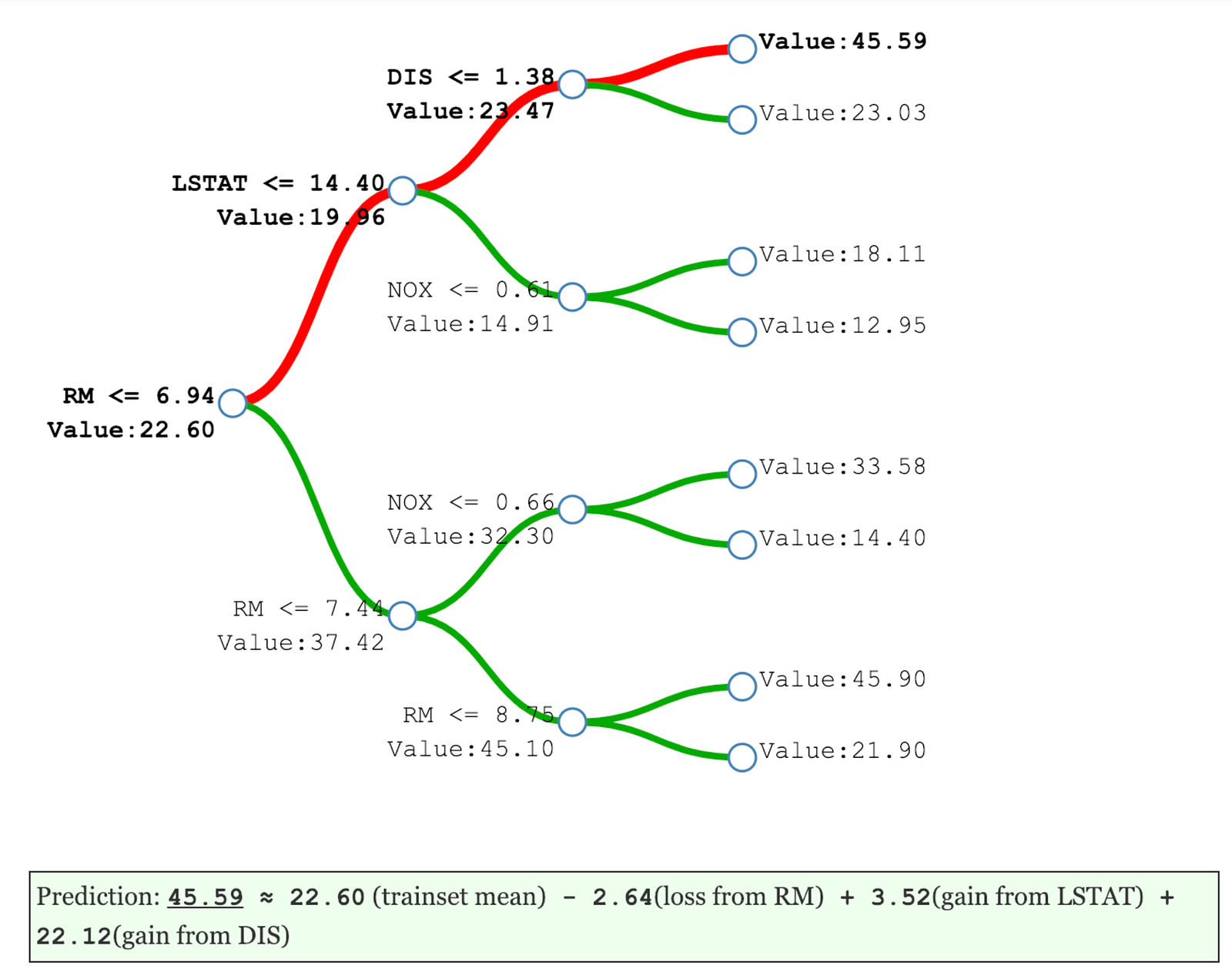As Random Forest’s prediction is the average of the trees, the formula for average prediction is the following: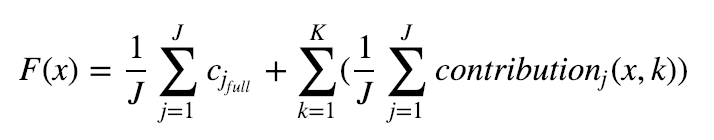where J is the number of trees in the forest.

I start by identifying rows with lowest and highest absolute prediction error and will try to see what caused the difference.

```Index with smallest error: 31Index with largest error: 85
```

Using `treeintrerpreter` I obtain 3 objects: predictions, bias (average value of the dataset) and contributions.

For the observation with smallest error, the main contributor was `LSTAT` and `RM` (which in previous cases turned out to be most important variables). In the highest error case, the highest contribution came from `DIS` variable, overcoming the same two variables that played the most important role in the first case.

```Row 31Prediction: 21.996 Actual Value: 22.0Bias (trainset mean) 22.544297029702978Feature contributions:LSTAT 3.02RM -3.01PTRATIO 0.36AGE -0.29DIS -0.21random 0.18RAD -0.17NOX -0.16TAX -0.11CRIM -0.07B -0.05INDUS -0.02ZN -0.01CHAS -0.01--------------------Row 85Prediction: 36.816 Actual Value: 50.0Bias (trainset mean) 22.544297029702978Feature contributions:DIS 7.7LSTAT 3.33RM -1.88CRIM 1.87TAX 1.32NOX 1.02B 0.54CHAS 0.36PTRATIO -0.25RAD 0.17AGE 0.13INDUS -0.03random -0.01ZN 0.0---------------------
```

To dive even deeper, we might also be interested in joined contribution of many variables (as explained in the case of XOR here). I will go right to the example, more information can be found under the link.

The most of the difference between the best and worst predicted cases comes from the number of rooms (`RM`) feature, in conjunction with weighted distances to five Boston employment centres (`DIS`).

### 2.2. LIME

LIME (Local Interpretable Model-agnostic Explanations) is a technique explaining the predictions of any classifier/regressor in an interpretable and faithful manner. To do so, an explanation is obtained by locally approximating the selected model with an interpretable one (such as linear models with regularisation or decision trees). The interpretable models are trained on small perturbations (adding noise) of the original observation (row in case of tabular data), thus they only provide good local approximation.

Some drawbacks to be aware of:

• only linear models are used to approximate local behaviour
• type of perturbations that need to be performed on the data to obtain correct explanations are often use-case specific
• simple (default) perturbations are often not enough. In an ideal case, the modifications would be driven by the variation that is observed in the dataset

Below you can see the output of LIME interpretation.

There are 3 parts of the output:
1. Predicted value
2. Feature importance — in case of regression it shows whether it has a negative or positive impact on the prediction, sorted by absolute impact descending.
3. Actual values of these features for the explained rows.

Note that LIME has discretized the features in the explanation. This is because of setting `discretize_continuous=True` in the constructor above. The reason for discretization is that it gives continuous features more intuitive explanations.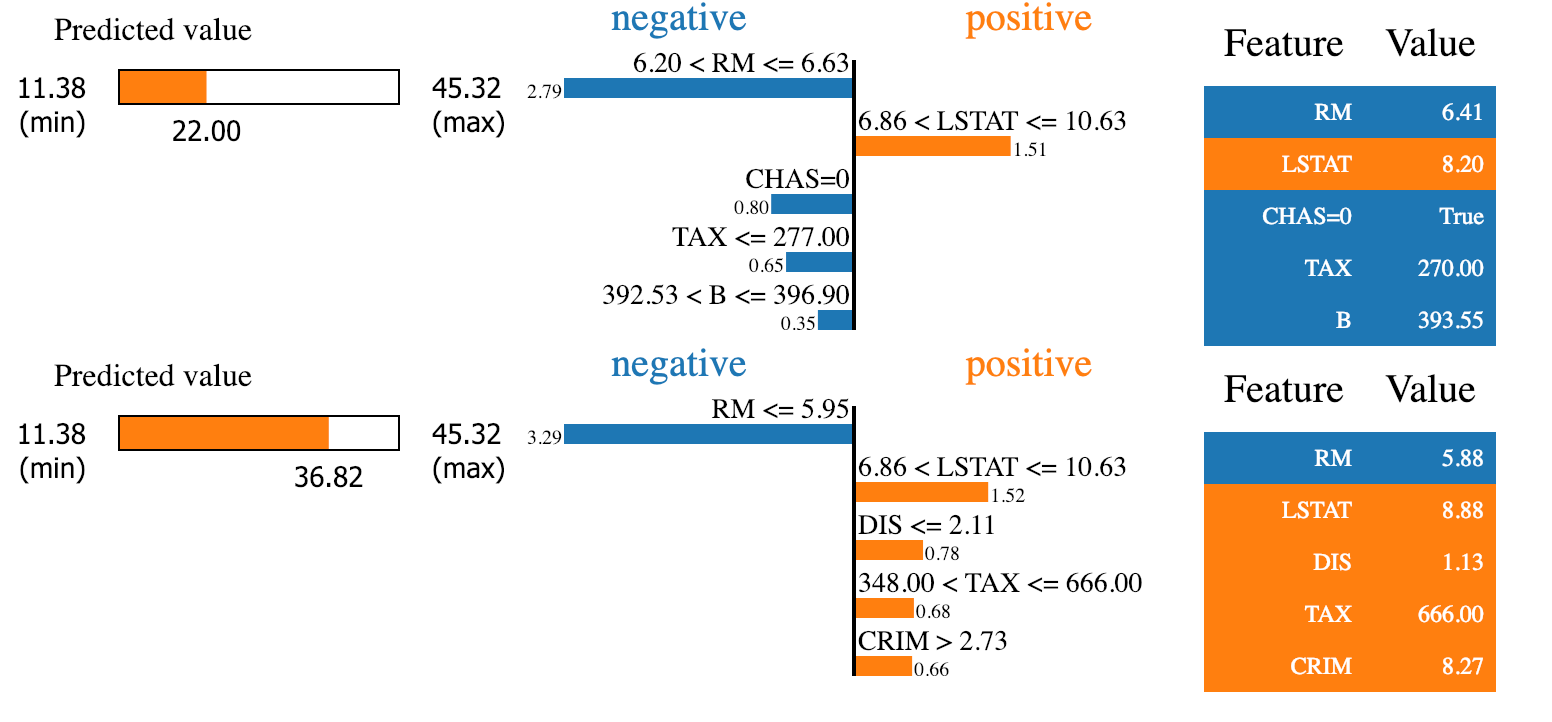LIME interpretation agrees that for these two observations the most important features are `RM` and `LSTAT`, which was also indicated by previous approaches.

### Conclusion

In this article I showed a few approaches to deriving feature importances from machine learning models (not limited to Random Forest). I believe that understanding results is often as much important as having good results, thus every data scientist should do his/her best to understand which variables are the most important for the model and why. Not only can this help getting a better business understanding, but also can lead to further improvements of the model.

As always, you can find the code used for this article on my GitHub. In case you have any questions or feedback, please let me know in the comments.